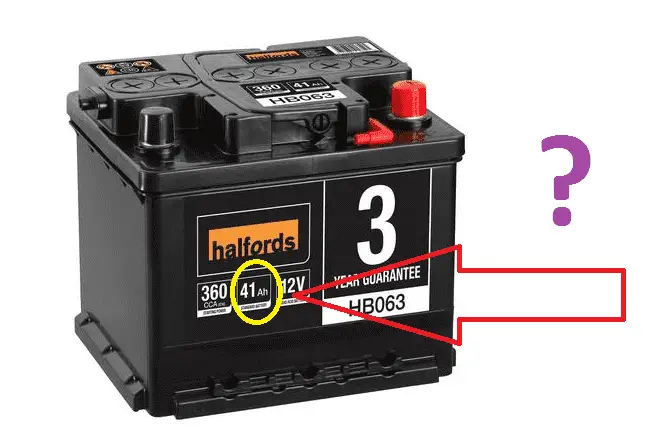# Why is Battery United AmpsHour AH

## Why is Battery United AmpsHour AH?

Battery is the device is used to store electrical energy in the form of chemical energy. Battery converts electrical energy into chemical energy. When we apply DC voltage across the battery terminal, the battery will charge, also we cannot store Alternating current.## What is AH (Amps hour):

AH is the unit of battery which means the battery is having the capacity to supply current for one hour without interruption or continuously.

For example, 1AH means, the battery supplies one ampere current for one hour for given rated voltage. An ampere is a unit of measure of the rate of electron flow or current in an electrical conductor. One ampere of current represents one coulomb of electrical charge moving past a specific point in one second.

• So we have another option (1C (Coulomb) = 6.25 x 10ˆ18 electrons, or 6,250,000,000,000,000,000 electrons.
• In addition, 1A (Ampere) = 1 coulomb of electrons per second and,
1h = 3600 Seconds
Therefore;
1Ah = (1A) x (3600s) = (C/s) x (3600s) = 3600 C.
∴ A (1 Ampere) = 1 Coulomb per second = C/s

## Why battery rating in AH?

• As we said above the battery is energy storing device, generally energy measured in Watt hour in DC. Hence in DC energy is measured in Wattshour. The output of the battery is equal to voltage * amps * time
• The amp-hour is a unit of battery energy capacity, equal to the amount of continuous current multiplied by the discharge time, that a battery can supply before exhausting its internal store of chemical energy.
• A battery with a capacity of 1 amp-hour should be able to continuously supply a current of 1 amp to a load for exactly 1 hour, or 2 amps for 1/2 hour, or 1/3 amp for 3 hours, etc., before becoming completely discharged.
• An amp-hour battery rating is only an approximation of the battery’s charge capacity and should be trusted only at the current level or time specified by the manufacturer. Such a rating cannot be extrapolated for very high currents or very long times with any accuracy.
• Discharged batteries lose voltage and increase in resistance. The best check for a dead battery is a voltage test under load.